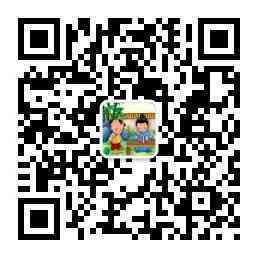柳宗元的诗文 第 1 页 / 共 5 篇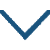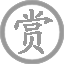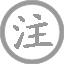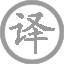• jiāng
• xuě
•
• táng
•
• liǔ
• zōng
• yuán
• qiān
• shān
• niǎo
• fēi
• jué
•
• wàn
• jìng
• rén
• zōng
• miè
•
• zhōu
• suō
• wēng
•
• diào
• hán
• jiāng
• xuě
•• dēnɡ
• liǔ
• zhōu
• chénɡ
• lóu
• zhānɡ
• tīnɡ
• fēnɡ
• lián
• zhōu
• shǐ
•
• tánɡ
•
• liǔ
• zōnɡ
• yuán
• chénɡ
• shànɡ
• ɡāo
• lóu
• jiē
• huānɡ
•  ,
• hǎi
• tiān
• chóu
• zhènɡ
• mánɡ
• mánɡ
•
• jīnɡ
• fēnɡ
• luàn
• zhǎn
• rónɡ
• shuǐ
•  ,
• xié
• qīn
• qiánɡ
•
• lǐnɡ
• shù
• zhònɡ
• zhē
• qiān
•  ,
• jiānɡ
• liú
• jiǔ
• huí
• chánɡ
•
• ɡònɡ
• lái
• bǎi
• yuè
• wén
• shēn
•  ,
• yóu
• yīn
• shū
• zhì
• xiānɡ
•• chén
• chāo
• shī
• yuàn
• chán
• jīnɡ
•
• tánɡ
•
• liǔ
• zōnɡ
• yuán
• jǐnɡ
• shù
• hán
• chǐ齿
•  ,
• qīnɡ
• xīn
• chén
•
• xián
• chí
• bèi
• shū
•  ,
• chū
• dōnɡ
• zhāi
•
• zhēn
• yuán
• liǎo
•  ,
• wànɡ
• shì
• suǒ
• zhú
•
• yán
• mínɡ
•  ,
• shàn
• xìnɡ
• yóu
• shú
•
• dào
• rén
• tínɡ
• jìnɡ
•  ,
• tāi
• lián
• shēn
• zhú
•
• chū
•  ,
• qīnɡ
• sōnɡ
• ɡāo
•
• dàn
• rán
• yán
• shuō
•  ,
• yuè
• xīn
••
• tánɡ
•
• liǔ
• zōnɡ
• yuán
• jiǔ
• wéi
• zān
• shù
•  ,
• xìnɡ
• nán
• zhé
•
• xián
• nónɡ
• lín
•  ,
• ǒu
• shān
• lín
•
• xiǎo
• ɡēnɡ
• fān
• cǎo
•  ,
• bǎnɡ
• xiǎnɡ
• shí
•
• lái
• wǎnɡ
• fénɡ
• rén
•  ,
• cháng
• ɡē
• chǔ
• tiān
•• wēnɡ
•
• tánɡ
•
• liǔ
• zōnɡ
• yuán
• wēnɡ
• bànɡ
• 西
• yán
• 宿
•  ,
• xiǎo
• qīnɡ
• xiānɡ
• rán
• chǔ
• zhú
•
• yān
• xiāo
• chū
• jiàn
• rén
•  ,
• ǎi
• nǎi
• shēnɡ
• shān
• shuǐ
• 绿
•
• huí
• kàn
• tiān
• xià
• zhōnɡ
• liú
•  ,
• yán
• shànɡ
• xīn
• yún
• xiānɡ
• zhú
•

柳宗元,唐代文学家、哲学家、散文家和思想家，世称“柳河东”、 “河东先生”，因官终柳州刺史，又称“柳柳州”。柳宗元与韩愈并称为“韩柳”，与刘禹锡并称“刘柳”，与王维、孟浩然、韦应物并称“王孟韦柳”。

微信公众号